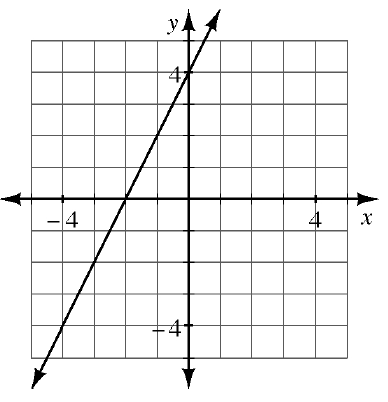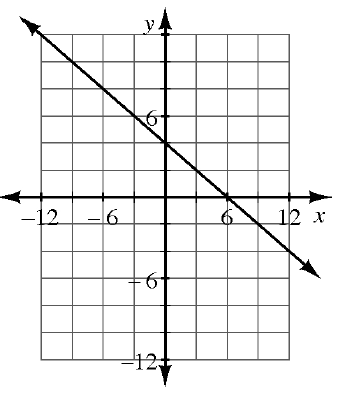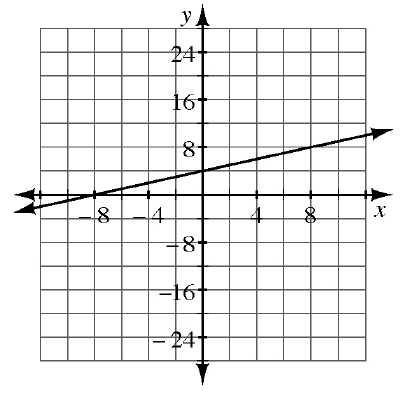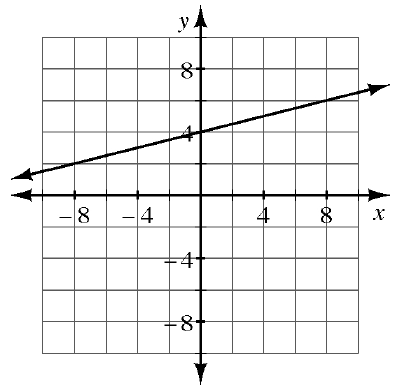### Home > CCAA8 > Chapter 2 Unit 2 > Lesson CCA: 2.2.3 > Problem2-74

2-74.

MATCH-A-GRAPH

Match the following graphs with their equations. Pay special attention to the scaling of each set of axes. Explain how you found each match.

Find the corresponding graph for each equation by starting with the $y$-intercept, then use the slope to find the next point.

1. $y=\frac{1}{4}x+4$

1. $y=\frac{1}{2}x+4$

1. $y=2x+4$

1

1. $y=-\frac{2}{3}x+4$

2

1.1.1.1.